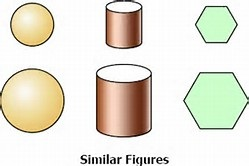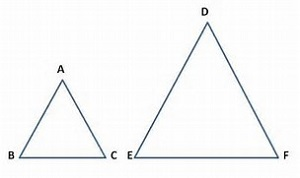# Triangles class 10 Notes Mathematics## myCBSEguide App

CBSE, NCERT, JEE Main, NEET-UG, NDA, Exam Papers, Question Bank, NCERT Solutions, Exemplars, Revision Notes, Free Videos, MCQ Tests & more.

CBSE class 10 Mathematics Chapter 6 Triangles notes in PDF are available for free download in myCBSEguide mobile app. The best app for CBSE students now provides Triangles class 10 Notes latest chapter wise notes for quick preparation of CBSE board exams and school based annual examinations. Class 10 Mathematics notes on Chapter 6 Triangles are also available for download in CBSE Guide website.

## CBSE Guide Triangles class 10 Notes

CBSE guide notes are the comprehensive notes which covers the latest syllabus of CBSE and NCERT. It includes all the topics given in NCERT class 10 Mathematics text book. Users can download CBSE guide quick revision notes from myCBSEguide mobile app and my CBSE guide website.

## 10 Mathematics notes Chapter 6 Triangles

Download CBSE class 10th revision notes for Chapter 6 Triangles in PDF format for free. Download revision notes for Triangles class 10 Notes and score high in exams. These are the Triangles class 10 Notes prepared by team of expert teachers. The revision notes help you revise the whole chapter in minutes. Revising notes in exam days is on of the the best tips recommended by teachers during exam days.

CBSE Class 10 Mathematics
Revision Notes
CHAPTER 06
TRIANGLES

1. Similar Figures
2. Similarity of Triangles
3. Criteria for Similarity of Triangles
4. Areas of Similar Triangles
5. Pythagoras Theorem
6. Miscellaneous Questions

1. Similar Figures: Similar figures have the same shape (but not necessarily the same size). In geometry, two squares are similar, two equilateral triangles are similar, two circles are similar and two line segments are similar.2. Similar Triangles: Two triangles are said to be similar if their corresponding angles are equal and their corresponding sides are proportional.

3. Equiangular Triangles: Two triangles are equiangular if their corresponding angles are equal. The ratio of any two corresponding sides in two equiangular triangles is always the same.

4. Criteria for Similarity:
in and(i) AAA Similarity:   when ,  and

(ii) SAS Similarity: , when  or   or  and

(iii) SSS Similarity: , when

4. The proof of the following theorems can be asked in the examination:

(i) Basic Proportionality Theorem: If a line is drawn parallel to one side of a triangle to intersect the other sides in distinct points, the other two sides are divided in the same ratio.
(ii) Converse of Basic Proportionality Theorem: If in two triangles, the corresponding angles are equal, their corresponding sides are proportional and the triangles are similar.
(iii) If one angle of a triangle is equal to one angle of other triangle and the sides including these angles are proportional, the triangles are similar.
(iv) If a perpendicular drawn from the vertex of the right angle of a right triangle to the hypoenuse, the triangles on each side of the perpendicular are similar to the whole triangle and to each other.
(v) Area Theorem: The ratio of the areas of two similar triangles is equal to the square of the ratio of their corresponding sides.
(iii) Pythagoras Theorem: In a right triangle, the square of the hypotenuse is equal to the sum of the squares of the other two sides.
(iv) Converse of Pythagoras Theorem: In a triangle, if the square of one side is equal to the sum of the squares of the other two sides then the angle opposite to the first side is a right angle.

## Triangles class 10 Notes

• CBSE Revision notes for Class 10 Mathematics PDF
• CBSE Revision notes Class 10 Mathematics – CBSE
• CBSE Revisions notes and Key Points Class 10 Mathematics
• Summary of the NCERT books all chapters in Mathematics class 10
• Short notes for CBSE class 10th Mathematics
• Key notes and chapter summary of Mathematics class 10
• Quick revision notes for CBSE board exams

## CBSE Class-10 Revision Notes and Key Points

Triangles class 10 Notes. CBSE quick revision note for Class-10 Mathematics, Chemistry, Maths, Biology and other subject are very helpful to revise the whole syllabus during exam days. The revision notes covers all important formulas and concepts given in the chapter. Even if you wish to have an overview of a chapter, quick revision notes are here to do if for you. These notes will certainly save your time during stressful exam days.

To download Triangles class 10 Notes, sample paper for class 10 Mathematics, Social Science, Science, English Communicative; do check myCBSEguide app or website. myCBSEguide provides sample papers with solution, test papers for chapter-wise practice, NCERT solutions, NCERT Exemplar solutions, quick revision notes for ready reference, CBSE guess papers and CBSE important question papers. Sample Paper all are made available through the best app for CBSE students and myCBSEguide website.Test Generator

Create question papers online with solution using our databank of 5,00,000+ questions and download as PDF with your own name & logo in minutes.